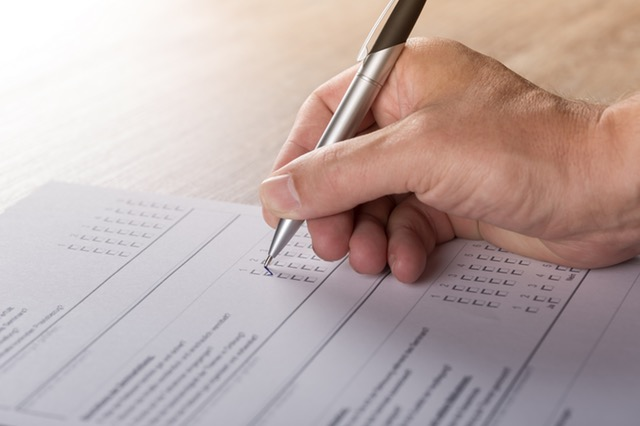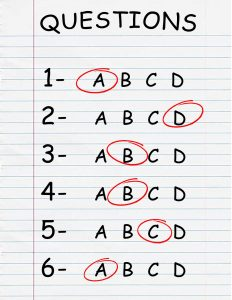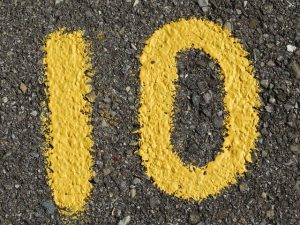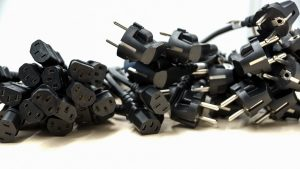# GMAT Math Tricks: The 9 Best Tips and ShortcutsAs you may already know, the math required for the GMAT Quant section is actually fairly basic: nothing beyond early high school-level math is tested. What’s challenging is how quickly you’ll need to be able to execute calculations to finish within the 62 minutes allotted for the 31 questions and the reasoning and analysis required to get to the right answer. In other words, the GMAT Quant section, like all other sections of the test, is more a test of how you think than what you know.

Luckily, this means that there are several GMAT math tricks, tips, and shortcuts that you can use to improve your performance. In this post, we’ll give you all the major GMAT quant tricks, including tips and shortcuts for each of the two question types as well as some that apply to both. With these GMAT math tricks in your arsenal—plus the boatloads of studying you’re surely doing—you’ll be well prepared to nail the Quant section on test day.

## GMAT Math Tricks: What Can They Help You With?

The makers of the GMAT will tell you that there are no such things as “tips” or “tricks” for doing well on the Quant section. Unfortunately, there is some truth to this: while GMAT math tricks can help you a little bit, the only real way to ace the GMAT Quant Section is to invest lots of time in focused, targeted preparation. Yep, that means study, study, study.

That said, each of the GMAT quant tricks below are extremely useful. Some help you execute basic calculations (like multiplication and division with unwieldy numbers) more quickly and efficiently; some help you get to the right answer without even having to solve the equation. Additionally, many of these GMAT Quant tricks are particularly helpful for guessing strategically on questions you’re stuck on—so when all else fails, you can feel like you have a solid plan and a fighting chance to get the right answer.General GMAT math tricks apply to all question types.

Not sure how or what to study? Confused by how to improve your score in the shortest time possible? We've created the only Online GMAT Prep Program that identifies your strengths and weaknesses, customizes a study plan, coaches you through lessons and quizzes, and adapts your study plan as you improve.

We believe PrepScholar GMAT is the best GMAT prep program available, especially if you find it hard to organize your study schedule and don't want to spend a ton of money on the other companies' one-size-fits-all study plans.## General GMAT Math Tricks

Below are some overall GMAT Quant tricks—as in, tips and shortcuts that apply to both question types.

### #1: Simplify Calculations with Multiples of 10

Working with multiples of 10 is easy in addition, subtraction, multiplication and division. Even when you’re given a less pretty number, you can still use multiples of 10 to solve it painlessly.

#### Addition and Subtraction with 10

To add two and three digit numbers that aren’t already a multiple of 10 or 100, round the number to the nearest 10s or 100s digit, do the addition, and then add or subtract the result by the number you rounded off. Do the opposite when subtracting.

\$\$525 + 311\$\$
\$\$= 500 + 311 + 25\$\$
\$\$= 811 + 25\$\$
\$\$= 836\$\$

#### Multiplying and Dividing with 10 via the Distributive Property

To multiply by an awkward number, such as 16, you can multiply first by 10, and then multiply by 6, and then add the two products together:

\$\$n × 16 = (n × 10) + (n × 6)\$\$

You may remember that the rule that applies to this calculation is called the distributive property, and it works as follows:

\$\$a (b+c) = (a × b) + (a × c)\$\$

This works for subtraction as well:

\$\$a (b – c) = (a × b) – (a × c)\$\$

Here’s a full example:

\$\$5 × 37 = 5 × (40 – 3)\$\$
\$\$= (5 × 40) – (5 × 3)\$\$
\$\$= (200) – (15)\$\$
\$\$= 185\$\$

The distributive property is one of the most helpful tools in your GMAT toolkit because it simplifies unwieldy calculations.The number 10 is easy to work with, as we use a base 10 math system.

#### Multiplying and Dividing between 11 and 19 via 10

There’s a slightly different trick for multiplying any two numbers between 11 and 19. Here, you can add the ones digit of one number to the other number, multiply that result by 10, and then add the product of just the ones digits.

Here’s an example:

\$\$11 × 17 = (18 × 10) + (1 × 7) = 187\$\$

#### Squaring between 11 and 19 via 10

To square any number \$n\$ between 11 and 99, find the nearest multiple of 10, and then find out how much you would have to add or subtract to get there. We’ll call the value that you’d have to add or subtract to get to a multiple of 10 \$d\$, for “difference.”

Next, do the opposite function with \$d\$ and the original number \$n\$ (add it if you had to initially subtract it to get to a multiple of 10, subtract if you had to add) to get two numbers that average out to \$n\$ (\$n\$ + \$d\$ and \$n\$ – \$d\$).

Finally, multiply those two numbers and add the square of \$d\$.

Here’s an example:

\$\$57^2 = (60 × 54) + 3^2\$\$
\$\$57^2 = (60 × 54) + 9\$\$
\$\$57^2 = (10 × 6 × 54 ) + 9\$\$
\$\$57^2 = 3240 + 9 = 3249\$\$

Want to improve your GMAT score by 60 points?

We have the industry's leading GMAT prep program. Built by Harvard, MIT, Stanford, and Wharton alumni and GMAT 99th percentile scorers, the program learns your strengths and weaknesses and customizes a curriculum so you get the most effective prep possible.Little GMAT math tricks like the above make mental math faster and easier, which is key to success on the Quant section, as you don’t have access to a calculator.GMAT math tricks aren’t quite like magic tricks, but they’re close!

### #2: Plug in Numbers, but With Care

Many GMAT Quant questions don’t require you to solve all of the many equations embedded within them. Sometimes picking a simple number and substituting it for the unknown variable works even better—and makes the problem simpler and easier—than actually solving the complex algebraic equation.

For problem solving questions—especially when you’re looking for a rate, ratio, fraction, or percentage of an unknown whole—picking a value to stand in for the unknown can save time and make it much easier to visualize and solve the problem.

Here’s an example problem solving question that shows this strategy in action:

To practice law in their state, the third year law students at Western University have to pass the bar examination. If ⅓ of the class opted not to take the bar examination and ¼ of those who did take the test, did so and failed. What percent of the 3Ls will be able to practice law in their state?

This question provides a perfect use case for plugging in numbers. Since we’re dealing with ⅓ and ¼, you should choose a number that both 3 and 4 factor into neatly. So let’s go with 12, the lowest common multiple.

If the class has 12 people in it and ⅓ don’t take the test, that means 4 don’t take it and 8 do. Of those 8 who did take the test, 2 fail, so 6 in total are able to practice. The answer is asking for the percentage, which is now easy: 6/12 is ½, or 50%.

For data sufficiency questions, however, plugging in numbers is only really helpful for proving that a statement is insufficient. The reverse is much more time consuming, and so it doesn’t make sense over solving the problem, so only resort to it if you strongly suspect that a given statement isn’t sufficient and you don’t know another way to proceed.

Here’s an example of this strategy in action in a data-sufficiency-style question (with just one statement, for the purposes of illustrating number-picking):

If integer \$n\$ is greater than 1, is 2\$n\$ – 1 prime?
1) \$n\$ is even

For this first statement, let’s plug in some even values for \$n\$:

Try \$n\$ = 2. We get 2\$n\$ – 1 = 22 – 1 = 3, and 3 is prime.

Want to Identify YOUR GMAT Strengths and Weaknesses?

Our proprietary GMAT Diagnostic Assessment creates a customized study plan for you that takes you from registration all the way to test day! It is included with every account and proven to significantly maximize your score.

Get your personalized assessment as part of your 5 day risk-free trial now:So far so good, but now try \$n\$ = 4. We get 2\$n\$ – 1 = 24 – 1 = 15, and 15 is not prime. So statement 1 is not sufficient.Be careful how you PLUG in numbers—you don’t want to end up with a jumbled mess of calculations!

#### 4 Tips for Picking Numbers

Here are four tips for picking numbers effectively and avoiding common traps in both question types:

1. Make sure that the number you pick meets all of the conditions in the question.

Note that some of the conditions may not be stated in their “official” terms, so you’ll have to read into the given information to recognize the rules at play. For example, a question stem might tell you that a given number y “has only two factors.” This means that y has to be a prime number. This is yet another example of how the math itself isn’t all that hard on the GMAT—what’s hard is uncovering the buried information that the question stem is masking by putting it in unusual words.

2. Be careful to avoid making assumptions beyond the given conditions.

For example, if your question states that \$a\$, \$b\$, and \$c\$ are consecutive numbers, you can’t then assume that \$a\$<\$b\$<\$c\$ or that \$a\$>\$b\$>\$c\$. All you know is that they are consecutive—you don’t know the exact order in which they each occur.

Another example is if the question states that \$x\$ > 5. Many would assume that the number has to be 6 or higher. But unless it is stated that \$x\$ must be an integer or a whole number, then we can’t make this assumption, as there are an infinite number of decimal values between 5 and 6.

Assuming that the answer is a whole number without being told is a mistake people make all the time on the GMAT.

3. Avoid a number that represents a possible exception to the general rules of a condition.

For example, 2 is the only even prime number and can lead to some confounding results when worked with in an equation, so you may not want to choose it as your “plug-able” number in a prime numbers question.

4. Plug in numbers that are easy to work with.

Don’t use a crazy number like 367—the whole point is to make the problem simpler! As long as they meet all the rules of the conditions given (and don’t have their own confounding special properties), simple numbers like 3, 4, 5, etc. should be fine.Be sure to plug in nice, easy numbers that will result in neat equations.

## GMAT Data Sufficiency Tricks

Data sufficiency questions are tough for everyone at first, since they’re stylistically different from the math problems you’re used to doing. Once you get used to them, however, you can discern some tricks and shortcuts that are baked into the unique format of these peculiar questions. Below are the best GMAT data sufficiency tricks.

### #1: Work Methodically Through the Choices

With their unchanging list of answer options, data sufficiency questions lend themselves perfectly to a special kind of process of elimination: You should always work through the answer choices in the same order.

We’ve pasted the choices below for your review. As soon as possible, you should memorize these answer choices until you know them cold—this will save you a good deal of time on the test. Note that they won’t come with A-E lettering on the real thing (we’ve put that in to make referring to them easier); instead, they’ll each have a bubble to the left that you’ll click on to indicate the answer.

A. Statement (1) ALONE is sufficient, but statement (2) alone is not sufficient to answer the question asked.

B. Statement (2) ALONE is sufficient, but statement (1) alone is not sufficient to answer the question asked.

C. BOTH statements (1) and (2) TOGETHER are sufficient to answer the question asked, but NEITHER statement ALONE is sufficient to answer the question asked.

D. EACH statement ALONE is sufficient to answer the question asked.

E. Statements (1) and (2) TOGETHER are NOT sufficient to answer the question asked, and additional data specific to the problem are needed.

First: test statement 1. If it isn’t sufficient to find one and only one answer, then eliminate (A) and (D). If it is sufficient, eliminate (B), (C), and (E).

Next, test statement 2. If it isn’t sufficient and statement 1 was sufficient, then (A) is the answer. If it is sufficient and statement 1 was also sufficient, then (D) is the answer. If it is sufficient and statement 1 wasn’t sufficient, then (B) is the answer.

If it isn’t sufficient and statement 1 also wasn’t sufficient, then either (E) or (C) is the answer.

You should only put the statements together if, after testing each statement for sufficiency by itself and going through the process of elimination above, both statements are insufficient and you’re left with (E) and (C). At this point, there are only two options: either they’re sufficient when taken together, or they’re not. If putting them together gets to only one answer, then (C) is the answer. If not, then (E) is the answer.Good thing there’s only (A) through (E) on the GMAT!

### #2: You Don’t Always Have to Solve Questions All the Way

Very often, you don’t necessarily have to determine the value of the expression in the data sufficiency question prompt or in the statements: Your task is simply to determine if the information provided is each statement is enough to do so.

For example, let’s say you get the DS question stem: “What is the value of \$x\$?” (This is a fairly common type of DS stem.) And let’s say you’re given the following in statement 1:

Statement 1: 22\$x\$ + 251 = 550

Being the studious person that you are, you might be tempted to solve for \$x\$ in statement 1 by subtracting 251 from both sides, and then solving for \$x\$ by dividing by 22 (which is tricky, since it doesn’t result in a whole number). But you don’t actually need to solve for \$x\$: Just by looking at the equation, you know that statement 1 can lead to only one possible value for \$x\$, so it is sufficient to determine the value of \$x\$, as asked.

Already, you can eliminate (B), (C), and (E)—and you didn’t even need to “do” any math!You don’t always have to find the exact value of a given variable in a data sufficiency question.

### #3: Use the “\$n\$ Variables, \$n\$ Equations” Rule

Data sufficiency questions that ask you to solve for one variable often feature two variables (usually \$x\$ and \$y\$) in the statements. Remember the “\$n\$ variables, \$n\$ equations” rule of linear equations for these questions: you need \$n\$ distinct equations to solve for \$n\$ variables; thus to solve for \$x\$ and \$y\$, you need two distinct equations that include both \$x\$ and \$y\$.

This means that statement 1 alone is usually not sufficient, so you can eliminate (A) and (D) after a quick glance to make sure that’s true. However, don’t just pick (C) and move on: You must simplify each equation to double-check that one isn’t the same as the other.

Here’s a brief example:

What is the value of \$x\$?
Statement 1: 8\$y\$ = 12 – 4\$x\$
Statement 2: 2\$x\$ + 4\$y\$ = 6

Both of these equations are the same, so you can’t solve for one variable by plugging in its equivalent expression of the other:

\$x\$ + 2\$y\$ = 3
\$x\$ = (3 – 2\$y\$)
2(3 – 2\$y\$) + 4\$y\$ = 6
6 – 4\$y\$ + 4\$y\$ = 6
6 = 6
\$y\$ = ?

As long as there are two distinct equations with \$x\$ and \$y\$ (and there are no squared variables, which we’ll get into below), then both statements together should be sufficient

### #4: Avoid the Square Root Trap

If \$x\$2 is any positive number, then \$x\$ could be a positive number or a negative number, as a negative times a negative results in a positive as well. This means that there are two possible values for \$x\$, one negative and one positive. Assuming that “\$x\$2 = [any positive number]” provides sufficiency to get to one value of \$x\$ is a very common mistake, but it’s easy to avoid by simply remembering this rule!With a few key GMAT data sufficiency tricks, you can avoid the scenario above!

## GMAT Problem Solving Tricks

GMAT problem solving questions are often thornier than they appear. Below are the best GMAT math trick, tips and shortcuts to help you strategically approach even the toughest problem solving questions.

### #1: Look at All the Answer Choices Before Solving

This is generally a better strategy than to solve the problem right away and then look for a choice that matches your solution, as the choices themselves can provide clues to how to solve the problem—especially if there’s a property or shortcut that can help you do so. As always, the GMAT almost never requires you to do extremely laborious equations out by hand—they want to see that you can get to the right answer efficiently (as an excellent business person would)!

### #2: Estimate to Cross off Wildly Wrong Answers

On a related note, many problem-solving questions test your ability to approximate reasonably, rather than precisely solving a complicated equation.

For example, let’s say you have to multiply a given number by a strange fraction, such a 11/53. This is fairly close to ⅕, or .2. The GMAT wants you to get to the right answer efficiently: they don’t want you to do all the work of dividing the given number by 53 and then multiplying by 11 on your scratchpad. It’s highly likely that the wrong answer choices will be far away from about ⅕ of the number, with only one choice that’s even in the ballpark.

Alternatively, you may get a question that appears to ask you to multiply many large numbers together, but the answer choices are all in exponent form and are all an order of magnitude away. In this case, you might be able to just estimate and find the closest answer as well.

Here’s an example of a good problem solving question to use estimation on:

James Woods High School’s senior class has 160 boys and 200 girls. If 75% of the boys and 84% of the girls plan to attend beauty school, what percentage of the total class plan to attend beauty school?

A. 75

B. 79.5

C. 80

D. 83.5

E. 84

The first thing to note is that 84% is an unwieldy number. When you see figures like that, it’s a sign that you may want to look for an estimating shortcut.

So what can we eyeball? Since there are 20% more girls than boys, we know that the weighted average will be closer to the girls’ percent than the boys’ percent. So we should look at the answer choices to see what we can already get rid of.  We can easily eliminate A, since 75% is going to be too low to be the weighted average. We can also cross out D and E, since they will both be too high (and are essentially equal).

79.5%, as the unweighted average of 75% and 84%, is the low extreme—the right answer will be slightly higher than that when adjusted for the total number of boys and girls, but just by a little bit, since there’s not a drastic difference in number between the two groups. So C, at 80%, looks right just by estimating. C is in fact the correct answer.GMAT math tricks can help you guess strategically when you’re stuck, but they can’t help you guess where the ball in the cup is!

### #3: Backsolve

Rather than plugging in numbers of your own choosing, some problem solving questions can be solved by working backward: plug the answer choices in, do the equation(s) with them, and cross off the choices that don’t balance. Usually there’s a faster way to get to the right answer, but this method can be a lifesaver when you really just don’t know how else to solve a given question.

The best way to approach backsolving is to start with C: the value that’s in the middle of all the choices. This way, even if it doesn’t balance the equation, you can determine whether the number that will work will be higher or lower (and rule out the higher or lower answer choices accordingly).

Here’s an example of a problem solving question that lends itself to backsolving:

What is the smallest positive integer \$x\$ for which \$x\$3 + 5\$x\$ is more than 80?

A. 2
B. 3
C. 4
D. 5
E. 6

As always when backsolving, start with C, \$x\$ = 4.

\$\$4^3 + 5(4) = 64 + 20 = 84\$\$

This is just over 80, so C could be the answer and, even if not, we can already eliminate D and E. Our powers of estimation tell us that 2 (A) is definitely going to yield a result far below 80, so let’s just check that 3 (B) doesn’t work:

\$\$3^3 + 5(3) = 27 + 15 = 42\$\$

That’s below 80, so \$x\$ = 4 is the smallest possible integer that satisfies the condition and C is the answer.

## In Conclusion: Summary of GMAT Math Tricks

Simplify unwieldy calculations with the distributive property and other other tricks that allow you to do basic arithmetic with easy numbers like 10. Plugging in numbers is a great strategy for when you can’t think of another way to solve the equation, but be careful with the numbers that you choose. Plugging in numbers works best to prove insufficiency for data sufficiency questions, but it may make more sense to backsolve for problem solving questions.

You can “game” data sufficiency questions by working methodically through the answer choices, and remember that you don’t always have to solve or “do out” a complex equation to prove sufficiency. And if you encounter two variables on a data sufficiency problem, you will need two equations to solve for both of them.

Avoid traps by remember that positive squares can have positive or negative square roots, and by not presuming a number is an integer unless told.

For problem solving questions, look at the answer choices before solving the problem. Once you’ve identified what the question is asking of you, estimate to cross off answers that can’t possibly work. Sometimes estimating will get you all the way to the right answer. Finally, don’t be afraid to backsolve—but always start with C, or the middle value, when doing so.

## What’s Next?

Check out our guide to 10 tips to master the quant section.

When you’re ready to conquer the subject areas tested, check out our GMAT-specific guides to integer properties, geometry formulas, and rate problems.

If you need help getting started and developing a study plan, we’ve got you covered there too.

Happy prepping!